# The Benefits Of Low Correlation: Round II

October 21, 2008This article is an update of an article published in the November/December 2007 issue of the Journal of Indexes titled "The Benefits of Low Correlation." Please see the introduction and literature review of the original article.

Description And Justification
This study examines the aggregate correlation among various assets and the corresponding impact on portfolio performance as measured by standard deviation of annual returns, internal rate of return (IRR), worst one-year portfolio drawdown (or loss), worst three-year cumulative return, and frequency of portfolio loss. These last three measures will prove to be more compelling performance measures than standard deviation of return.

Worst one-year portfolio loss is a measure of the percentage change in the portfolio account value from one year to the next. When examining a withdrawal portfolio during retirement, this measure also takes into account the increasing annual withdrawals that occur at the end of each year. Frequency of portfolio loss is a measure of how often the portfolio lost value from year-end to year-end.

It is proposed that three measures of portfolio risk-worst one-year portfolio loss, frequency of loss and worst three-year cumulative return-are more intuitively useful (and compelling) to the average investor than is standard deviation of return.

Analysis
We first consider a fundamental difference between a buy-and-hold accumulation portfolio and a retirement withdraw-mode portfolio. As shown in Figure 1, the mathematics of recovery from a loss are much more severe in a retirement distribution portfolio than in a preretirement accumulation portfolio. For instance, if an accumulation portfolio sustains a 10 percent loss, it only needs a 3.6 percent average annualized return over the next three years to restore its pre-loss account balance. In a retirement distribution portfolio, the needed average annualized return to recover from a 10 percent loss within three years following the loss is 11.5 percent.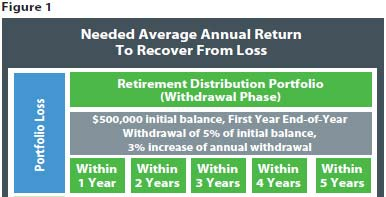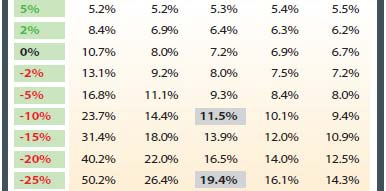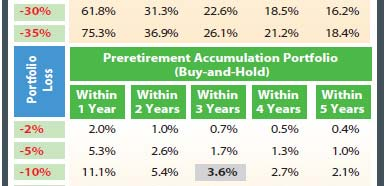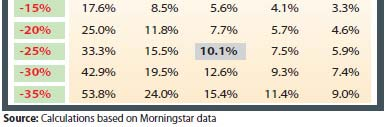If the loss is 25 percent, the preretirement accumulation portfolio must generate a 10.1 percent annualized return over three years, whereas the required annual return in a distribution portfolio is nearly double at 19.4 percent. Notice that a distribution portfolio is forced into "recovery mode"; even if the return that it is recovering from is positive (5 percent, 2 percent) or 0 percent. This is due to the fact that the required return must exceed the withdrawal rate that is increasing each year.

As clearly seen by the required returns to restore losses, recovery is considerably more challenging in a distribution portfolio. In light of that, avoiding large losses in a retirement portfolio is the highest priority-as demonstrated by the raw mathematics of recovery.

The time frame covered in this study was the 38-year period from 1970-2007. Assets included in this analysis were large-cap U.S. equities, small-cap U.S. equities, non-U.S. equities, U.S. intermediate-term bonds, cash, real estate and commodities (see Figure 2). The 38-year historical performance of large-cap U.S. equities is represented by the S&P 500 Index, while the performance of small-cap U.S. equities is captured by using the Ibbotson Small Company Index from 1970-1978, and the Russell 2000 Index from 1979-2007. The performance of non-U.S. equities was represented by the Morgan Stanley Capital International EAFE Index (Europe, Australasia, Far East) Index. U.S. intermediate-term bonds were represented by the Ibbotson Intermediate Term Bond Index from 1970-1976 and the Lehman Brothers Intermediate Government Bond Index from 1977-2007.

The historical performance of cash is represented by three-month Treasury Bills. The performance of real estate was measured by using the annual returns of the National Association of Real Estate Investment Trusts (NAREIT) Index (annual returns for 1970 and 1971 were regression-based estimates inasmuch as the NAREIT Index did not provide annual returns until 1972). Finally, the historical performance of commodities was measured by what is now known as the S&P GSCI.

The bivariate correlations between each asset are reported in Figure 2. For example, the 38-year average correlation between large U.S. equities and small U.S. equities was 0.739, or 73.9 percent. The correlation between U.S. intermediate-term bonds and commodities was -0.210. The aggregate correlation for the entire seven-asset portfolio (as calculated by computing the average of the 21 bivariate correlations) was 0.127.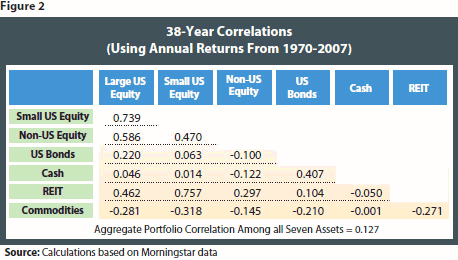Preretirement Accumulation Portfolio
The annual returns of each asset from 1970-2007 are provided in Figure 3, as well as the results for an accumulation portfolio that includes all seven assets (equally weighted and annually rebalanced to maintain the equal weighting). Data in red parentheses represent negative returns. The primary data source for this study was Morningstar Principia. Raw data were also obtained from "Stocks, Bonds, Bills, Inflation"; by Ibbotson Associates.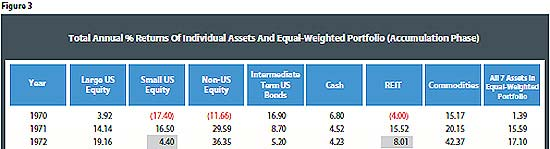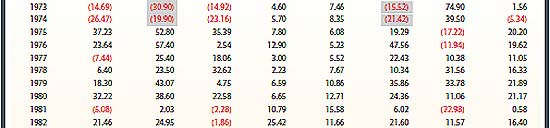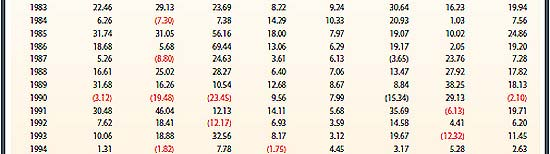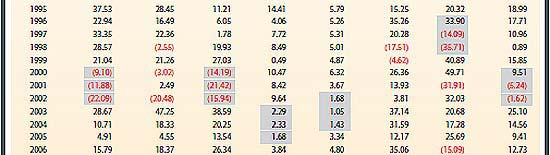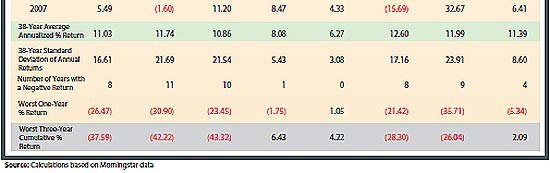Also shown in Figure 3 is the 38-year average annualized return for each individual asset, as well as the standard deviation of annual returns, the number of years with a negative one-year return, the worst one-year return and the worst three-year cumulative percentage return. All these figures assume a buy-and-hold investment. The period in which the worst three-year cumulative return occurred is shaded in yellow.

The equally weighted seven-asset portfolio (EW) generated performance that was comparable (or better) than the individual equity assets but with return volatility (standard deviation) only slightly higher than bonds. Its worst single-year loss was -5.34 percent compared with losses of over -20 percent for each of the individual equity assets. Impressively, the EW portfolio never had a three-year period with negative cumulative return.

Three additional portfolios are contrasted against the EW portfolio in Figure 4: a 60 percent equity/40 percent fixed-income moderate allocation portfolio, a custom-weighted seven-asset portfolio (CW) and a 40 percent equity/60 percent fixed-income conservative allocation portfolio. The 60/40 portfolio is typical among "moderate allocation"; funds, such as Vanguard Wellington or Dodge & Cox Balanced. The 40/60 portfolio is a common asset allocation model among "conservative allocation"; funds, such as Vanguard Wellesley Income or Franklin Income. The EW portfolio had an aggregate allocation of 42 percent equities, 29 percent fixed income and 29 percent in "alternative"; or diversifying equitylike assets. The CW portfolio allocation was 12 percent large U.S. equities, 8 percent small U.S. equities, 10 percent non-U.S. equities, 40 percent bonds, 20 percent cash, 5 percent REITs and 5 percent commodities.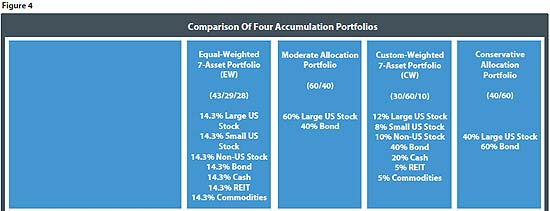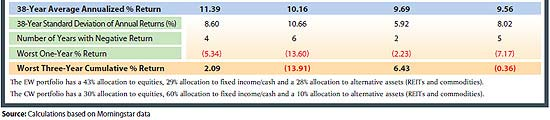The CW portfolio underperformed the EW portfolio by 170 bps, but volatility of return (as measured by standard deviation) was reduced by 268 bps. While a reduction in volatility is intuitively desirable, it is proposed that the other three measures in Figure 4 are more compelling to the average investor.

For instance, the CW portfolio had only two years with a negative return, whereas the EW portfolio had four years with a loss. The worst one-year return was only -2.23 percent for CW compared with -5.34 percent for EW. Finally, the worst three-year cumulative return in CW was 6.43 percent versus 2.09 percent in EW. It is instructive to note that the multi-asset portfolios (EW and CW) had significantly better downside protection than the two-asset portfolios (moderate 60/40 allocation and conservative 40/60 allocation). Downside protection is a direct benefit of assembling portfolios with more diverse assets.

The risk/return characteristics of the individual assets and the four portfolios are shown in Figure 1. The EW portfolio is shown by the beige square, CW is the orange square, the 40/60 portfolio is the plum square, and the 60/40 portfolio is the dark blue square. Each individual asset is shown by a different-colored dot.

As shown in the scatter graph, there is meaningful correlation between performance and risk. Risk is measured by worst three-year cumulative return. The individual assets (represented by different-colored dots) depict the classic upward sloping shape of the risk/return frontier. Assets that provide higher return potential tend to expose the investor to larger worst-case three-year losses. Asset allocations of 100 percent bonds or 100 percent cash eliminated large portfolio losses over any three-year period, but their performance was significantly lower than the individual equity assets and the four portfolios. Without sufficient growth, the likelihood of outliving one's retirement portfolio obviously increases.

The benefit of creating diversified portfolios is demonstrated by the location of the squares. For example, the seven-asset portfolios (beige square and orange square) eliminated negative returns over any three-year period during the 38-year time frame of this study. Importantly, the seven-asset portfolios avoided prolonged losses while maintaining a level of performance that was comparable (or superior) to most of the individual equity assets.

With this connection between performance and worst-case three-year cumulative return in mind, the information presented in Figure 1 is more persuasive. For example, a 25 percent loss in a distribution portfolio will require a 19.4 percent annual return over the subsequent three-year period to restore the loss compared with "only"; a 10.1 percent needed annual return over the next three years in an accumulation portfolio. It is important to note that five of the individual assets in this study had worst-case three-year cumulative losses in excess of -25 percent (large U.S. equities, small U.S. equities, non-U.S. equities, REITs and commodities). Indeed, three of the individual assets had worst-case three-year returns below -37 percent. (And this is assuming a buy-and-hold portfolio. In distribution portfolios, market losses are magnified because of money being withdrawn). Recovering from losses of this magnitude is far more problematic in a retirement portfolio than in a preretirement accumulation portfolio.

Postretirement Distribution Portfolio

In analyzing a retirement distribution portfolio, a starting balance of \$500,000 was assumed, with an initial withdrawal at the end of the first year of 5 percent of the starting portfolio balance (in this case, \$25,000), and an annual increase in the withdrawal of 3 percent to account for annual inflation. Thus, the second-year withdrawal in this analysis was \$25,750, the third-year withdrawal was \$26,523 and so forth.

As shown in Figure 6, the first distribution portfolio analyzed consisted of 100 percent cash. Understandably, this portfolio allocation may not represent a typical retirement portfolio. It is included in this analysis simply as a starting point. The 38-year IRR of the 100% cash portfolio was 7.04 percent with a standard deviation of 3.08 percent. The worst-case one-year portfolio drawdown (i.e., the percentage change in portfolio account value from year-end to year-end) was -13.9 percent, which occurred in 2007 (as the account value began to plummet). The frequency of loss for the all-cash portfolio (i.e., the percentage change in account value from year to year) was 53 percent -or in 20 out of the 38 years. In those 20 years, the average loss was -4.4 percent.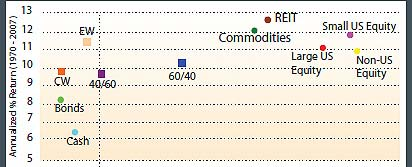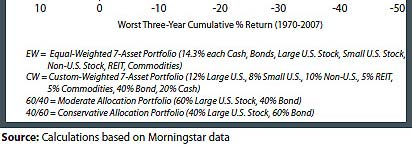Adding bonds to the cash portfolio (both equally weighted) improved the IRR to 7.71 percent but caused a slight increase in standard deviation (3.63 percent versus 3.08 percent in the all-cash portfolio). The aggregate correlation of the two-asset portfolio was 0.41. The worst-case one-year portfolio drawdown was dramatically reduced, from -13.9 percent to -3.4 percent. Moreover, the percentage of years with a loss was lowered from 53 percent to 24 percent, and the average annual loss was reduced from -4.4 percent to -2.0 percent. These improvements in the performance of the portfolio were not revealed by standard deviation-in fact, using only standard deviation of return as the measure of portfolio volatility would have completely missed the beneficial impact of adding a second asset to the portfolio.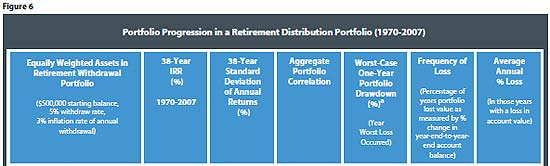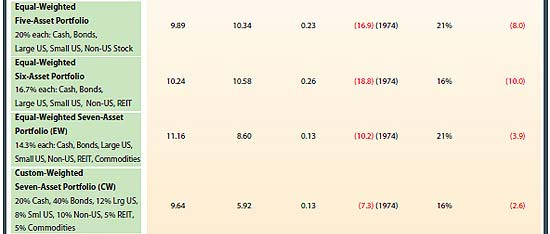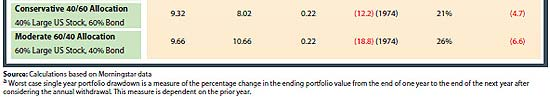Next, U.S. large-cap equities were added to the portfolio. The three assets (cash, bonds, large U.S. equities) were each equally weighted at 33.33 percent of the portfolio. With three assets, the aggregate correlation of the portfolio declined from 0.41 to 0.22. The 38-year IRR of the portfolio increased by 98 bps to 8.69 percent, but the standard deviation of return increased from 3.63 percent to 6.44 percent. The worst-case one-year portfolio drawdown increased to -9.4 percent. However, the three-asset portfolio only lost value 18 percent of the time, compared with 24 percent in the two-asset portfolio. Once again, standard deviation of return increases, but frequency of loss decreases as the aggregate portfolio correlation decreased.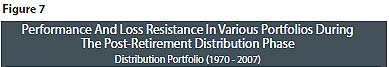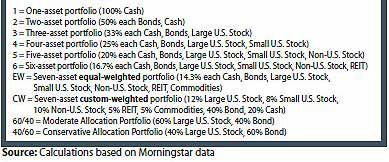When adding the fourth asset (small U.S. stocks), the aggregate portfolio correlation increased slightly to 0.25, portfolio return improved by 80 bps, standard deviation increased from 6.44 percent to 9.33 percent, worst one-year loss widened abruptly to -14.2 percent, frequency of loss increased slightly to 24 percent and the average loss increased from -4.3 percent to -5.3 percent. The inclusion of the fourth asset was beneficial to performance but detrimental to aggregate correlation, worst-case loss, frequency of loss and average loss. The reason for this is that small U.S. stocks have such a high correlation to large U.S. stocks (the prior asset added). Adding an asset that has a high correlation to an asset already in the portfolio is not nearly as helpful to the portfolio (in a holistic way) as adding an asset that has a low correlation. In this particular case, it lifted return but at the cost of greater loss exposure.

Adding non-U.S. stocks as the fifth asset (20 percent allocated to each asset) lifted performance by 40 bps while changing aggregate portfolio correlation very little. Standard deviation of return and worst one-year loss increased, as did the average one-year loss (to -8.0 percent from -5.3 percent in the four-asset portfolio). Same story as above, non-U.S. equity has fairly high correlation to U.S. equity.

The sixth asset added was REITs. The performance of the portfolio increased by 35 bps to 10.24 percent with standard deviation increasing only slightly. The aggregate portfolio correlation was 0.26 and the worst case one-year loss increased to -18.8 percent. Compared with the five-asset portfolio, the frequency of loss declined to 16 percent, but the average annual loss increased to -10.0 percent.

Commodities were added as the seventh asset, with each asset having a 14.3 percent allocation in the portfolio. Return increased by 92 bps to 11.16 percent and aggregate portfolio correlation dropped to 0.13. Standard deviation of return declined by nearly 200 bps and the worst case one-year loss was reduced from -18.8 percent to -10.2 percent. The portfolio's frequency of loss was 21 percent, and the average loss declined significantly to -3.9 percent. The impact of adding commodities is dramatic because it is an asset with low correlation to every other asset in the portfolio (refer back to Figure 2).

Also shown in Figure 6 are the results for a custom-weighted seven-asset portfolio (CW) which had a 20 percent allocation to cash, 40 percent to bonds, 12 percent to large U.S. equity, 8 percent to small U.S. equity, 10 percent to non-U.S. equity, 5 percent to REITs and 5 percent to commodities. The CW portfolio represents a conservative 40 percent equity/60 percent fixed income-with two 5 percent slices of the overall equity allocation being placed in low correlation "alternative"; assets (REITs and commodities). The CW portfolio is more likely to be implemented than the equally weighted seven-asset portfolio because a 28.6 percent allocation to REITs and commodities (in the equal-weighted portfolio) may be perceived as too risky.

The CW portfolio generated a 9.64 percent IRR, but had a low standard deviation of return of 5.92 percent. Its worst one-year loss was -7.3 percent, it had annual losses 16 percent of the time and the average annual loss was a mere -2.6 percent. Only the two-asset portfolio of cash and bonds had a lower average annual loss, but the two-asset portfolio only had a 7.71 percent IRR and experienced losses 24 percent of the time.

Finally, the performance metrics for a conservative two-asset 40/60 portfolio and a moderate two-asset 60/40 portfolio are reported. Both generate impressive returns, but with higher aggregate correlation. Worst-case one-year losses were higher than in either seven-asset portfolio, as was the frequency of loss and the average annual percentage loss.

The relationship between portfolio performance (IRR) and frequency of loss is depicted in Figure 2. The location of the all-cash portfolio at the far right is instructive. Even though cash always has positive nominal returns, as the sole asset in a retirement distribution portfolio, it exposes retirees to annual losses over 50 percent of the time. The seven-asset portfolios (EW and CW) and the six-asset portfolio (#6 dot) represent ideal allocation models for postretirement distribution portfolios given their location closest to the northwest corner of the graph.

Summary
Achieving low standard deviation of return is a worthy goal, but should not be the primary goal in an accumulation portfolio or a distribution portfolio. Rather, achieving low aggregate portfolio correlation should be the primary goal. In an accumulation portfolio, creating diverse seven-asset portfolios (equal-weighted or custom-weighted) was shown to produce superior risk-adjusted performance. Moreover, losses over any three-year period were eliminated in the EW and CW portfolios.

In a retirement distribution portfolio, this analysis has demonstrated that successively adding equity assets to a fixed-income portfolio causes standard deviation of return to increase, but also dramatically lowers the frequency of portfolio losses. Portfolio losses are hard to ignore, whereas standard deviation of return is a more abstract concept.

The performance of the distribution portfolio improved and the aggregate portfolio correlation generally declined or held steady as each additional asset was added. Performance and aggregate correlation improved dramatically when the seventh asset (in this case, commodities) was added. Of the assets analyzed in this study, commodities are the only asset class that has consistently negative correlations with all the other asset classes. Consequently, it is the low correlation of commodities that generated a host of portfolio benefits, rather than simply being the last asset added.

Considering all the measures in this analysis (IRR, standard deviation, aggregate portfolio correlation, worst-case one-year loss, frequency of loss and average percentage annual loss), the ideal postretirement distribution portfolio is the CW portfolio. The EW portfolio is the best allocation model during the preretirement accumulation phase.

As shown by the mathematics of recovery for portfolios in withdrawal mode, avoiding large losses is of paramount importance. Compared with a "classic"; conservative 40 percent equity/60 percent fixed-income portfolio, the CW portfolio had a 32 bps higher return, 210 bps lower standard deviation of return, lower aggregate correlation (0.13 versus 0.22), dramatically lower worst-case loss (-7.3 percent versus -12.2 percent), lower frequency of loss (16 percent versus 21 percent) and lower average annual loss (-2.6 percent versus -4.7 percent).

Achieving low correlation among the assets in a portfolio is the key to generating a multitude of benefits. However, it requires the use of several assets that have low correlation with each other. Some of these "low-correlation"; assets may not fit the traditional paradigm of assets to be included in a retirement portfolio. Some paradigms need to change.

References

Bernstein, W., 2001, The Intelligent Asset Allocator, McGraw Hill

Markowitz, H., 1991, Portfolio Selection, Blackwell Publishing